## 【活动公告】6.0服务器端午节活动系列

6.0 2803℃

1. 到 法兰城(238.97) 与 尹雷斯 对话，得到 找寻粽子的请求

2. 到 里谢里亚堡(44.87) 与 夏科芙 对话交出 找寻粽子的请求，得到 鐮刀

3. 到 芙蕾雅(352.382) 与 蘭提斯 对话交出 鐮刀，得到 艾草

4. 回到 里谢里亚堡 与 夏科芙 对话交出 艾草，得到 粽子的情報

5. 到 伊尔村(31.74) 与艾斯樵 对话交出 粽子的情報，得到 避暑的香包

6. 到 杰诺瓦镇(64.50) 与 收集香包的小茜 对话交出 避暑的香包，得到 饭团便当

7. 到 沙连娜(137.411) 与 小櫻 对话交出 饭团便当，得到 龙舟设计图

8. 將 龙舟设计图 交回到 杰诺瓦镇(64.50) 的 收集香包的小茜 ，得到 龙舟

9. 再將 龙舟 带到 伊尔村(31.74) 与 艾斯樵 对话得到 粽子

10. 最後带著 粽子 到 法兰城(238.97) 与 尹雷斯 得到 龙头（有几率获得永久头饰）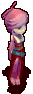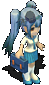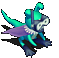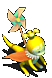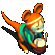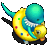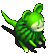充值后联系小宝领取

•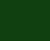2020-6-23
2
没有连战？
•2020-6-23
3
礼包也没有？
•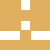2020-6-24
4

[寻找粽子]=

Point1=活动起点——法兰城

Point2=与尹雷斯对话，得到找 粽子的请求:238:98

Point3=入城堡:140:88

Point4=与夏科芙对话，得到 鐮刀:44:88

Point5=出城堡:64:53

Point6=出东门:280:88

Point7=与蘭提斯对话，得到 艾草 :352:383

Point8=回法兰:470:196:

Point9=入城堡:140:88

Point10=与夏科芙对话，得到 粽子的情報:44:88

Point11=出城堡:64:53

Point12=出东门:280:88

Point13=进伊尔村:682:343

Point14=与艾斯樵 对话，得到 避暑的香包 :31:73

Point15=出伊尔村:44:31

Point16=沙莲娜海底洞窟:200:165

Point17=沙莲娜海底1-2:19:8

Point18=沙莲娜海底2-1:10:9

Point19=沙莲娜海底1-莎莲娜:23:11

Point20=进杰诺瓦镇:216:456

Point21=与小茜 对话，得到 饭团便当:64:51

Point22=出杰诺瓦镇:25:40

Point23= 与 小櫻 对话，得到 龙舟设计图:137:412

Point24=进杰诺瓦镇:216:456

Point25=与小茜 对话，得到 龙舟:64:51

Point26=出杰诺瓦镇:25:40

Point27=莎莲娜-沙莲娜海底1:196:444

Point28=[反]沙莲娜海底1-2:13:41

Point29=[反]沙莲娜海底2-1:37:79

Point30=[反]沙莲娜海底1-芙蕾娅:21:33

Point31=进伊尔村:682:343

Point32=与艾斯樵 对话，得到 粽子 :31:73

Point33=出伊尔村:44:31

Point34=回法兰:470:196:

Point35=与尹雷斯对话，得到 龙头 :238:98

Point36=任务结束

Point37=端午安康,愿好人一生平安

Point38=资料来源:不死